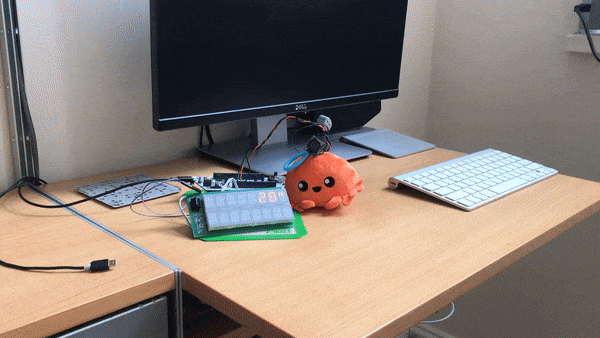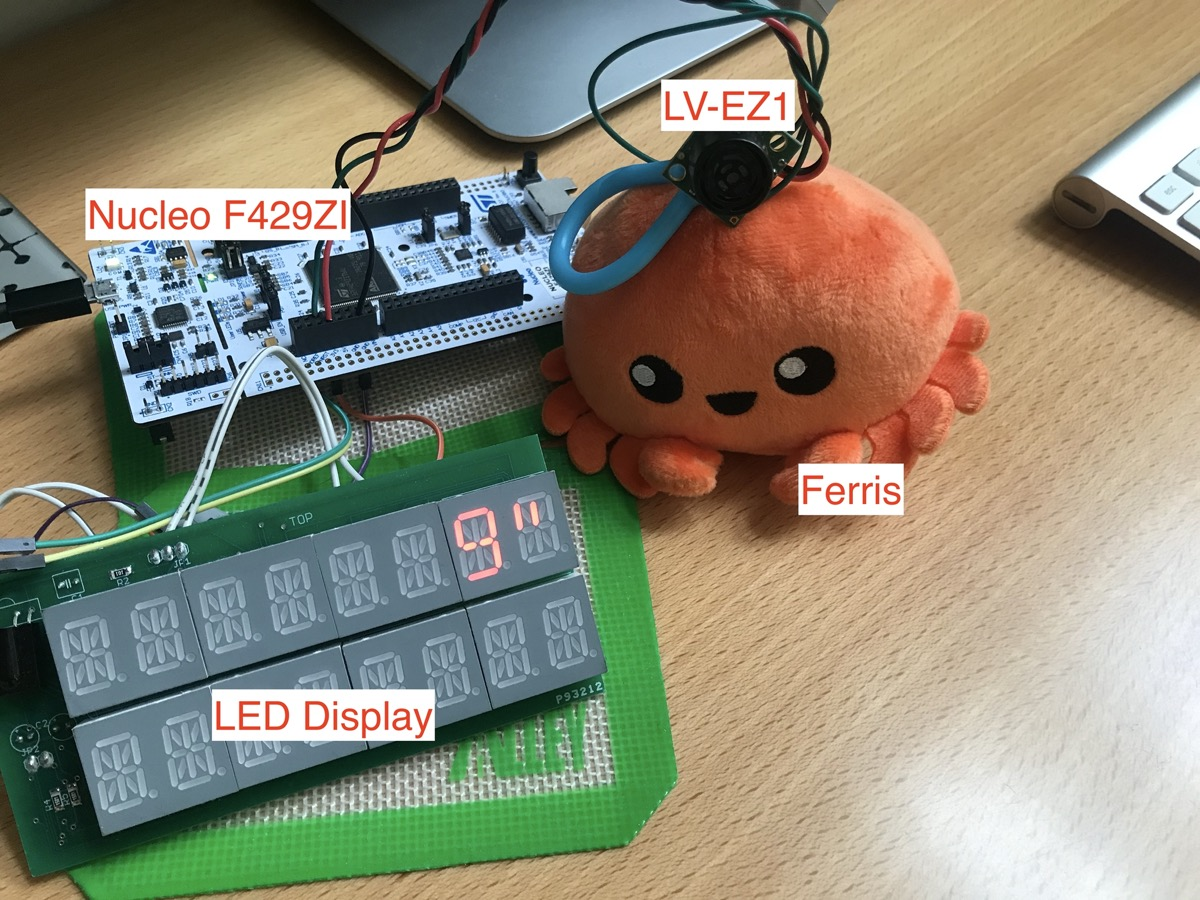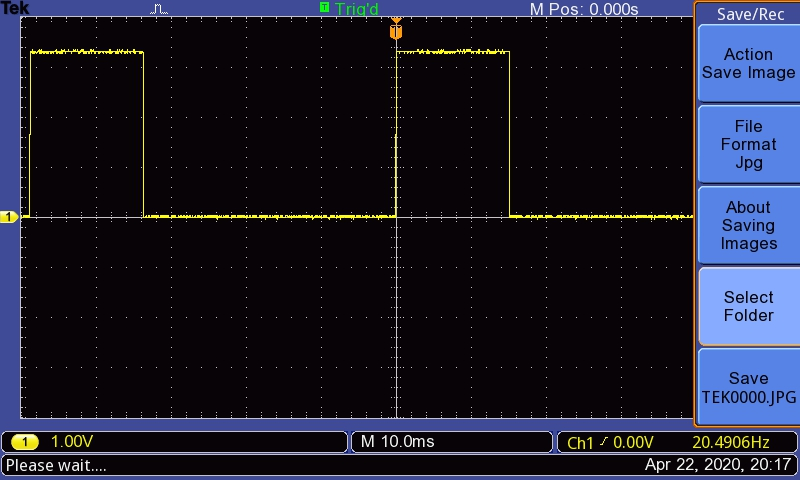This week, I played around with an ultrasonic range finder and wrote a little library for it in Rust. The outcome is shown in the image above. This system measures the distance between myself and Ferris.

I used an ultrasonic sensor from Maxbotix to measure distance. This project monitors the sensor’s output and shows the measured distance on a LED display.

Here are two new things I learned from this project:

• How to read microcontroller’s timer counter value
• How to convert `u32` (sensor reading) to `&str` (for the display) in a `no-std` environment where `format!` macro is not available

## Ingredients

### Hardware## Ultrasonic Sensor

Maxbotix ultrasonic sensor measures distance and outputs the reading through PWM or Analog. For this project, I read pulse width with a timer.

The output from the sensor looks like the screenshot below. The pulse width represents distance. You can learn more about pulse width based distance calculation at Maxbotix website.Reading the pulse width is straightforward. Basically, I observe the state of the input pin and read the timer counter value. When `read` method is called, it goes like this:

• Wait while the pin is low
• Reset the counter
• Wait while the pin is high
• Read the counter value and return it to the caller

## Implementation

### Three Sensor Models

The sensor comes in 3 models: LV, XL, and HR. They all work the same way. Just different resolutions. To calculate the distance, we use the model-specific scale factor.

• LV: 147uS/inch
• XL: 58uS/cm
• HR: 1uS/mm

I define an enum called `Model` like this:

``````impl Model {
/// scale factor
fn factor(self) -> u32 {
match self {
Model::LV => 147,
Model::XL => 58,
Model::HR => 1,
}
}
/// unit
fn unit(self) -> &str {
match self {
Model::LV => "\"",
Model::XL => "cm",
Model::HR => "mm",
}
}
}
``````

### Distance measurement using timer counter

I wrote a module called `maxsonar` to measure the pulse width by reading a timer’s counter value.

Here is the `struct` for the sensor. It takes a Timer (concrete `TIM2` of the HAL crate), `Model`, and generic `T: InputPin`.

``````pub struct MaxSonar<T> {
timer: TIM2,
model: Model,
pin: T,
}

impl<T, E> MaxSonar<T>
where
T: InputPin<Error = E>,
E: core::fmt::Debug,
{
pub fn new(timer: TIM2, model: Model, pin: T, sysclk: Hertz) -> Self {
// Configure timer for 1Mhz
let rcc = unsafe { &(*RCC::ptr()) };
rcc.apb1enr.modify(|_, w| w.tim2en().set_bit());
let psc = (sysclk.0 / 1_000_000) as u16;
timer.psc.write(|w| w.psc().bits(psc - 1));
timer.egr.write(|w| w.ug().set_bit());
// Start MaxSonar
let mut sonar = MaxSonar { timer, model, pin };
sonar.start();
sonar
}

fn start(&mut self) {
self.timer.cnt.reset();
self.timer.cr1.write(|w| w.cen().set_bit());
}
}
``````

It looks like `stm32f4xx-hal` doesn’t implement a method to read the current timer value. So, I directly access `TIM2`’s `cnt` register in my `read` method.

``````pub fn read(&mut self) -> u32 {
while self.pin.is_low().unwrap() {}
self.timer.cnt.reset();
while self.pin.is_high().unwrap() {}
}
``````

As you can see, I do these:

• Wait while the pin is low
• Reset the counter
• Wait while the pin is high

I then calculate the distance by dividing the counter value by the scale factor. `self.model.factor()` returns the scale factor of the chosen model. In this project, I use `Model::LV`. So, the factor is `147`.

### format! in a no-std environment

Great. I now have the distance as `u32`. I just need to convert it to `&str` to show it on my LED display. Here is the definition of the display driver’s `write_str` method:

``````pub fn write_str(&mut self, text: &str) -> Result<(), E>
``````

In a `std` environment, I would just call `format!` to make a `&str`. But, apparently I cannot do that in a `no-std` environment. After a struggle to find a way to convert `u32` to `&str`, I managed to achieve that with `heapless` crate. With `heapless::String` and `write!` macro, I can create formatted texts.

``````use core::fmt::Write;
use heapless::consts::*;
use heapless::String;

let mut data = String::<U8>::new();

loop {
`sonar.unit()` returns the unit for the chosen model. So, it is `"` here.